# NCERT Solutions for Class 4 Maths How Heavy How Light

NCERT solutions for Class 4 Mathematics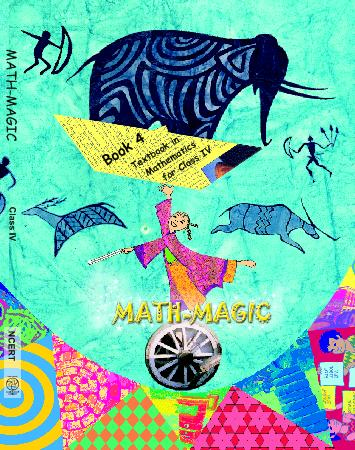## NCERT Solutions for Class 4 Maths How Heavy How Light

Chapter -12 Class 4 Maths How Heavy How Light

### 1. Find out the total weight they had loaded on the cart.

Ans. Let us find the total weight loaded on the cart.

 Thing Loaded Weight 5 sacks of wheat 5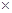100 kg = 500 kg 3 sacks of rice 335 kg = 105 kg A water tank = 50 kg An almirah = 70 kg 3 tables 310 kg = 30 kg 4 chairs 45 kg = 20 kg 2 mattresses 220 kg = 40 kg Bamboo ladder = 10 kg Pots and pans = 10 kg Total weight = 853 kg

Thus, the total weight loaded on the cart is 835 kg.

### 2. Which things should be removed so that the weight of the load is not more than 700 kgs?

Ans. Three sacks of rice and three table may be removed so that the weight of the load is not more than 700 kgs.

### 3. Now you also make your own balance. Write down how you made it. Also draw a picture of your balance in the box below.

Ans. To make a balance we need a uniform stick of suitable length, two pans and a thread to tie pans to the stick. It is made as shown in the below figure.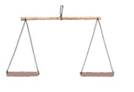### 4. Make pairs of different things and use the balance to decide which is heavier. First guess which thing will take the pan down and then check with your balance.

Ans. Consider the following pairs of things:

(i) toothbrush and toothpaste tube

(ii) spoon and cup

(iii) rubberband and pencil

In the first pair toothpaste tube is heavier, in the second pair cup is heavier, and in the third pencil is heavier.

### 5. Make groups of three things. For example- eraser, ball and paper. Use the balance to arrange them in order of weight- the lightest, the one with in between weight, the heaviest. Complete the table with at least five examples.

Ans.

 Lightest Paper In between weight Eraser HeaviestBall Napkin Scarf Shawl Grape Date Potato Orange Coconut Pumpkin Bottle Lemon Papaya

### 6. Can you find your own weight using this balance?

Ans. I cannot find my own weight using this balance.

Ans. 237 g.

### 8. Practice Time:

(a) Is the weight on any of the pans equal to 1 kilogram? Mark it.

Ans. In the above balance the pan containing the weight equal to 1 kilogram is right marked.

(b) How many grams are there in 1 kg?

Ans. 1 kg contains 1000 grams.

### 9. Name 5 things that we usually buy.

Ans.

 In grams In kilograms Camphor Wheat Sandal Rice Chilli powder Sugar Cloves Potato Cardamom Apple

### 10. Which is heavier- one kilogram cotton or one kilogram iron?

Ans. The weight of 1 kg cotton and 1 kg iron is equal.

### 11. Guess and write the weight of each thing be bought in g or kg.

Ans. After writing the weight in g or kg, the table is as under:

 Items Weight Rice 5 kg Sugar 1 kg Mustard Seeds 10 kg Wheat 3 kg Dal 500 g Tea 250 g Pepper 25 g

### 12. Car and tractor:

(a) Ritu is weighing her toys. She wants to know if her tractor is heavier than her car. How would you help her to find it quickly.

Ans. In the balance, place car in one pan and tractor in the other pan. Pan containing tractor goes down. So the tractor is heavier than car.

(b) Guess which is the heaviest- a real car, a bus or a tractor?

Ans. Of a real car, a bus or a tractor, a bus is the heaviest.

(c) Which is the heaviest thing you have seen?

Ans. The heaviest thing I have seen is a ship.

### 13. Now imagine what happened next and complete the story. Discuss with your friends how Vaidika’s daughter found he weight of the elephant.

Ans. The story is completed as under:

After the marking of the raise water level on the boat, the elephant was taken out of the boat. Now she requested to put stones into the boat till the water level touched the raised water level mark. Further she asked to weigh the stones. The total weight of these stones gave the weight of the elephant. King was not left with any alternative but to reward Dr. Vaidika.

### 14. Anamika wants to weigh this chair using the weight machine. Can you suggest a way for doing this?

Ans. In a balance, find the equivalent weight of chair by putting the chairs in one pan and stones in the other pan. Now put these stones on the platform of the weighing machine and and see the reading. This gives the weight of the chair.

### 15. Now you show how Abdul will see these stones pieces to weigh-

Ans.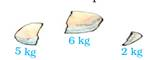### 16. (a) 4 kg of firewood?

Ans. By placing a bundle of wood and a stone weighing 2 kg in one pan and by placing a stone of weighing 6 kg in the other pan.

(b) 3 kg of firewood?

Ans. By placing a bundle of firewood and a stone weighing 2 kg in one pan and a stone weighing 5 kg in the other pan.

(c) 7 kg of firewood?

Ans. (C) By placing firewood in one pan and two stones of weight 2 kg and 5 kg in the other pan.

### 16. Post Office:

(a) Have you ever been to a post office?

Ans. Yes, I have been to a post office.

(b) What different things do people go there for?

Ans. People go there for purchasing postal items, posting letters/ parcels and banking etc.

(c) How much a postcard cost?

Ans. (c) A postcard costs 50 paise.

(d) How much an inland letter cost?

Ans. (d) An inland letter costs Rs. 2.50.

### 17. How much will you have to pay for stamps on a letter weighing 50 grams?

Ans. Charges for sending a letter weighing 50 grams:

Charges for 1 st 20 grams or less = Rs. 5.00

Charges for next 20 grams   = Rs. 2.00

Charges for next 10 grams   = Rs. 2.00

Total charges   = Rs. 9.00

Class 4 Maths How Heavy How Light

### 18. Akash wants to send a parcel of the Math Magic textbook to his friend Rani in Chennai. The book weighs 200 g. See the chart to find the cost of posting the book.

Ans. Charges for the book weighing 200 grams:

Charges for parcel weighing 50 grams = Rs. 5.00

Charges for next 150 grams at the rate of Rs. 3.00

For every additional 50 grams = Rs. 33.00 = Rs. 9.00

Total Charges = Rs. 14.00.

### 19. Read the weight shown in the picture. Find the cost of sending a parcel of that weight.

Ans. Parcel weight = 225 grams

Cost of stamps = Rs. 17.00

Clearly, the parcel weigh 225 grams.

Parcel Charges:

For first 50 gm   = Rs. 5.00

For next 150 gm    = Rs. 9.00

For next 25 gm   = Rs. 3.00

Total Charge = Rs.14.00

### 20. Rahul nees a stamp of Rs. 25 for his parcel. He went to the post office. Only stamps of Re.1, Rs. 2, Rs. 5 and Rs. 10 were there at that time. Using those stamps, in how different stamps, in how many different ways can he made Rs. 25? Can you show five different ways? What is the heaviest parcel he can seen using stamps of Rs. 25?

Ans. There are many ways to make Rs. 25 stamps using stamps of Re. 1, Rs. 2, Rs. 5 and Rs. 10. Some of these are as under:

(1) 25Re. 1

(2) 12Rs. 2+ 1Re. 1

(3) 5Rs. 5

(4) 2Rs. 10 + 1Rs. 5

(5) 2Rs. 10 + 5Re. 1

(6) 2Rs. 10+ 2Rs.2 + 1Re. 1

(7) 1Rs. 10 + 3Rs.5 etc.

### 21. What happened after that? So what was the answer the crow wanted to give?

Ans. The moment the crow opened his beak, the frog escaped. The crow wanted to give (650 + 145) g = 795 g as his answer.

Class 4 Maths How Heavy How Light

### 22. Now, you also fill the table by finding out the age, height and weight of any five friends.

Ans.

 Name Age Height Weight Sameer 8 4 feet 1 inches 21 kg Neetu 8 3 feet 11 inches 18 kg Amit 9 4 feet 10 inches 25 kg Megha 7 3 feet 10 inches 17 kg Jagmohan 10 4 feet 4 inches 24 kg

### 23. All oranges have equal weight. The two papayas have the same weight. The weight in the first and second balances are equal. How many oranges balance the weight in the third?

Ans. Let M, O and P represent mango, orange and papaya respectively.

In the first balance:

M+O = O + O + O

Or M + O – O = O + O+ O -O

Or M = O + O

M = 2 O ……(i)

In the second balance:

P + P = O + O + M

2P = 2O + M

2P = 2O + 2O   [using (i)]

P = 2 O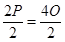…………(2)

In the third balance:

M + P = 2 O + 2 O

M + P = 4 O

Thus, 4 oranges will balance the weight in the third balance.

Class 4 Maths How Heavy How Light

### 24. There are 3 marbles of the same size but one marble is slightly heavier or lighter than the other two. Can you find which is that marble and if it is heavier or lighter?

You can use a balance only two times.

Ans. Let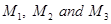be three marbles such that one of them is slightly heavier or lighter than the other two. Put marbles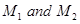in different pans. Two situations arise:

Case I: If the two pans balance each other, the marble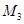is either heavier or lighter. This completes first weighing. In order to check whetheris heavier or lighter, take marble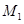and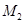. The position of pan containinggives the required result. It his pan goes down, thenis lighter thanand. Otherwise,is lighter thanand. Thus, the result is known in the second weighing.

Case II: If the two balances do not balance each other. Let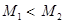. Takeand. On comparing their weights by balance, either.

(i)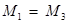, thenis heavier than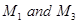.

(ii)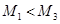then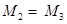andis lighter than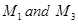.

Result: (i) and (ii) can be judged in the second weighing.

## NCERT solutions for Class 4 Mathematics Chapter 12 How Heavy How Light

Building with BricksView Solutions
Long and ShortView Solutions
A trip to BhopalView Solutions
Tick Tick TickView Solutions
The Way the World LooksView Solutions
The Junk SellerView Solutions
Jugs and MugsView Solutions
Carts and WheelsView Solutions
Halves and QuartersView Solutions
Play With PatternsView Solutions
Table and SharesView Solutions
How Heavy How LightView Solutions
Fields And FencesView Solutions
Smart ChartsView Solutions

NCERT Solutions Class 4 Maths How Heavy How Light PDF (Download) Free from myCBSEguide app and myCBSEguide website. Ncert solution class 4 Maths includes text book solutions from Class 4 Maths Book . NCERT Solutions for CBSE Class  Maths have total 14 chapters. 4 Maths NCERT Solutions in PDF for free Download on our website. Ncert Maths class 4 solutions PDF and Maths ncert class 4 PDF solutions with latest modifications and as per the latest CBSE syllabus are only available in myCBSEguide.

## CBSE app for Students

To download NCERT Solutions for Class 4 Maths, EVS Hindi English, Maths Science do check myCBSEguide app or website. myCBSEguide provides sample papers with solution, test papers for chapter-wise practice, NCERT solutions, NCERT Exemplar solutions, quick revision notes for ready reference, CBSE guess papers and CBSE important question papers. Sample Paper all are made available through the best app for CBSE students and myCBSEguide website.Test Generator

Create question paper PDF and online tests with your own name & logo in minutes.myCBSEguide

Question Bank, Mock Tests, Exam Papers, NCERT Solutions, Sample Papers, Notes

### 4 thoughts on “NCERT Solutions for Class 4 Maths How Heavy How Light”

1. Hehrfdhagdfeewretgggqdhf

2. Thank you so much????

3. This is so useful I like this app very much

4. best app and correct answers